§ 3  Special solutions to algebraic equations

Abel proved that there are no algebraic solutions to general equations of degree 5 and higher . But Abel's theorem does not answer the question: is there an algebraic solution to each given specific equation . Galois proved that there is an algebraic solution A specific integer-coefficient algebraic equation that cannot be solved by the method . For example

x 5 - x +1=0

Galois also found necessary and sufficient conditions for equations to be solved by radicals .

1. Find rational roots

According to the properties of "whole roots and rational roots" in the previous section, the rational roots of some specific equations can be found .

Find the rational roots of an equation .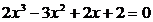Solution Since p and q of the rational roots of this equation are both divisors of 2 , they are 1 , -1 , 2 , and -2 . So the possible values ​​are 1 , -1 , 2 , and -2 . Divide by synthesis ( See § 2 , a )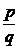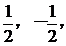test:

1) 2    - 3     2      2             - 1) 2 -  3       2      2

2-1    1-2 5-7 _ _ _ _ _ _2     - 1    1      3                 2    - 5      7     - 52    - 3     2      2 2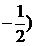- 3      2      21    - 1- 1      2     - 2

2     - 2    1 2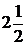- 4      4      0

So it is a rational root of the known equation .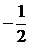Divide the original formula by , get the quotient formula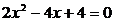which is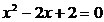The tower top discriminant 4 - 8<0 , its two roots are a pair of conjugate complex roots . Therefore, the original equation has only one rational root .2. Solve three equations

Shaped like

au 2 n + bu n +c =0

The equation of is called a three-term equation, where a, b, c, n are not equal to zero , n is an integer . It can be solved by radicals . Let u n = x , the quadratic equation ax 2 + bx+c =0.

Solve the equation by example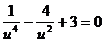Solve the order , then get , its root is and . From get . So. Substitute into the original equation to check, we can see that these four numbers are the roots of the equation .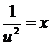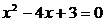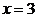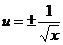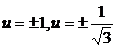3. Solve the reciprocal equation

Shaped like

ax n + bx n1 + cx n2 + L + cx 2 + bx + a =0

(where the coefficients of the xn -k and xk terms are the same) is called the reciprocal equation . Any root of the reciprocal equation is not equal to zero .

Divide both sides of the 1° even-numbered ( n = 2 k ) reciprocal equation by x k , and then set z = x + , the original equation can be transformed into a k -th equation of z , solve this equation, get the value of z , and then the corresponding x The value can be determined by the quadratic equation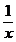x 2 - zx +1=0

Solution of odd-numbered ( n = 2 k +1) reciprocal equations boils down to solving even-ordered reciprocal equations .

Solve the equation by example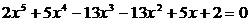The solution is a root of the original equation, and the equation is divided by the 4th reciprocal equation: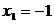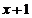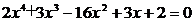Divide it by , and then combine, we get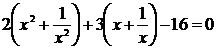Let , then , the above formula becomes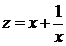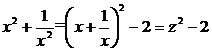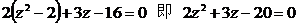From this, there are two definite equations: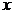and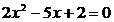From this we get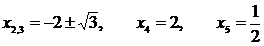4. Solve the binomial equation

In the form of x n - A =0

The equation of is called a binomial equation . Its n roots are the nth roots of the complex number A.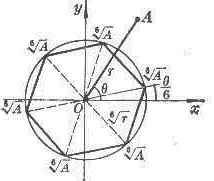If A is written as

A = r ( cos θ + i sin θ )

Then the n roots of the equation x n - A = 0 are( k = 0 , 1, 2 , L , n - 1)

Geometric Explanation: The points on the complex plane that correspond to the nth root of the number r ( cos θ + i sin θ ) are the vertices of a regular n - gon , and these vertices are on a circle with the origin as the center and the radius . And One of the vertices of this n -gon has an argument . Figure 3.1 shows the case of n = 6 .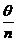If A =1 , then the solution ξ of x n =1 is called n -th unit root . The n n - th unit roots are

cos + i sin ( k = 0,1,2, L , n - 1) Figure 3.1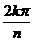If ξ is one of the n -th unit roots, then the n n - th unit roots are 1, ξ , ξ 2 , L , ξ n - 1 , which are geometrically represented as vertices of an inscribed regular n -gon of the unit circle .